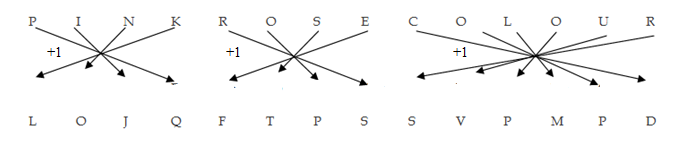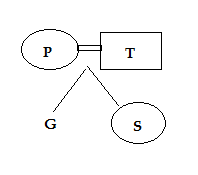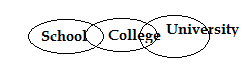# RRB ALP 2018 Practice Test Papers | Reasoning Questions (Day-84)

Dear Aspirants, Here we have given the Important RRB ALP & Technicians Exam 2018 Practice Test Papers. Candidates those who are preparing for RRB ALP 2018 can practice these Reasoning Questions to get more confidence to Crack RRB 2018 Examination.

[WpProQuiz 3207]

Click “Start Quiz” to attend these Questions and view Solutions

1) There are some Girls and Cows at a place. If total number of heads is 6 and total number of legs is 20, how many girls and how many cows are there?

a) 4 girls and 2 cows

b) 3 girls and 4 cows

c) 2 girls and 3 cows

d) 2 girls and 4 cows

2) If A =1, BAT = 5, then GANIT = ?

a) 2

b) 7

c) 6

d) 5

3) Which of the following word cannot be formed by this word “TRIANGLE”?

a) ALTERING

b) RELATING

c) INTEGRAL

d) ENTREATY

4) There are five friends A, B, C, D and E are sitting in a bench in the way of A is sitting 2nd right of B and second left of C. E is sitting second to the right of D and immediate neighbour of C. Who is sitting fourth from the right end?

a) A

b) B

c) C

d) D

5) If PINK is coded as LOJQ, ROSE is coded as FTPS, the COLOUR is coded as

a) SVPMPD

b) SPMVPD

c) SVDMPR

d) SMDPRP

6) P is Mother of G and wife of T. If S is daughter of T, then how is G related to S?

a) Brother

b) Sister

c) Either a) or b)

d) Cannot be determined

7) Father: Son : : Seed:

a) Leaves

b) Plant

c) Flower

d) Fruit

8) 91: 117:: 161:

a) 210

b) 219

c) 207

d) 234

9) If FACE is coded as 2134, PACE is coded as 5134, how is PEACE coded in that code?

a) 54323

b) 54134

c) 45123

d) 12234

10) Which of the following conclusion follow the given statement?

Some Schools are college

Some colleges are university

a) Some Schools are university

b) No university is Colleges

c) Some college is School

d) Some university is School

Girl =x Cow = y

x + y = 6          ————— (1)

2x + 4 y = 20      ————— (2)

Solving (1) & (2)

y = 4; x =2

A= 1, B =2, C = 3 and so on

BAT = 2 + 1 + 20 = 2 + 1+ 2 + 0 = 5

GAINT =7 + 1 + 9 + 14 + 20 = 7 + 1 + 9 + 1 + 4 + 2 + 0 = 6

They are sitting in the order of

B          D         A         E          CG is either Sister or Brother.

13*7 : 13 * 9 : : 23 * 7 : 23 * 9 = 91 : 117  : : 161 : 207